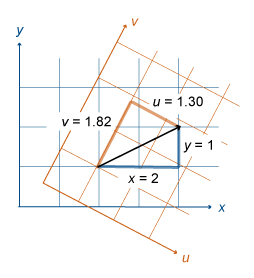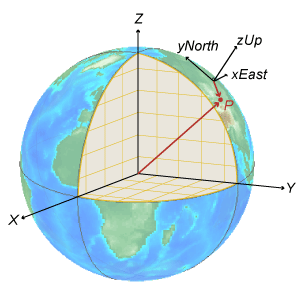## Vectors in 3-D Coordinate Systems

Vectors represent quantities such as velocity and acceleration. Mapping Toolbox™ functions transform vector components between Earth-centered Earth-fixed (ECEF) and east-north-up (ENU) or north-east-down (NED) systems. For more information about ECEF, ENU, and NED coordinate systems, see Choose a 3-D Coordinate System.

Unlike coordinates that measure position, vector components in a Cartesian system do not depend on a position in space. Therefore, when you transform a vector from one system to another, only the components of the vector change. The magnitude of the vector remains the same.

For example, this image shows a 2-D vector transformation from an x-y system to a u-v system. The vector has components x = 2 and y = 1 in the x-y system, and components u = 1.30 and v = 1.82 in the u-v system. The components of the vector are different, but in each system the magnitude of the vector is 2.24 units.This image shows a coordinate transformation from a global ECEF system to a local ENU system using `ecef2enu`. The position vectors start at the origin of each system and end at point P. Therefore, the transformation changes the magnitude of the position vector.This image shows a vector transformation from a global ECEF system to a local ENU system using `ecef2enuv`. The vector r does not depend on a position. Therefore, the transformation changes the components of the vector, but the magnitude of the vector is the same.### Tips

Unlike coordinate transformation functions such as `ecef2enu`, vector transformation functions such as `ecef2enuv` do not require you to specify a reference spheroid or the ellipsoidal height of the local origin. The geodetic latitude and longitude of the local origin is sufficient to define the orientation of the uEast, vNorth, and wUp axes.